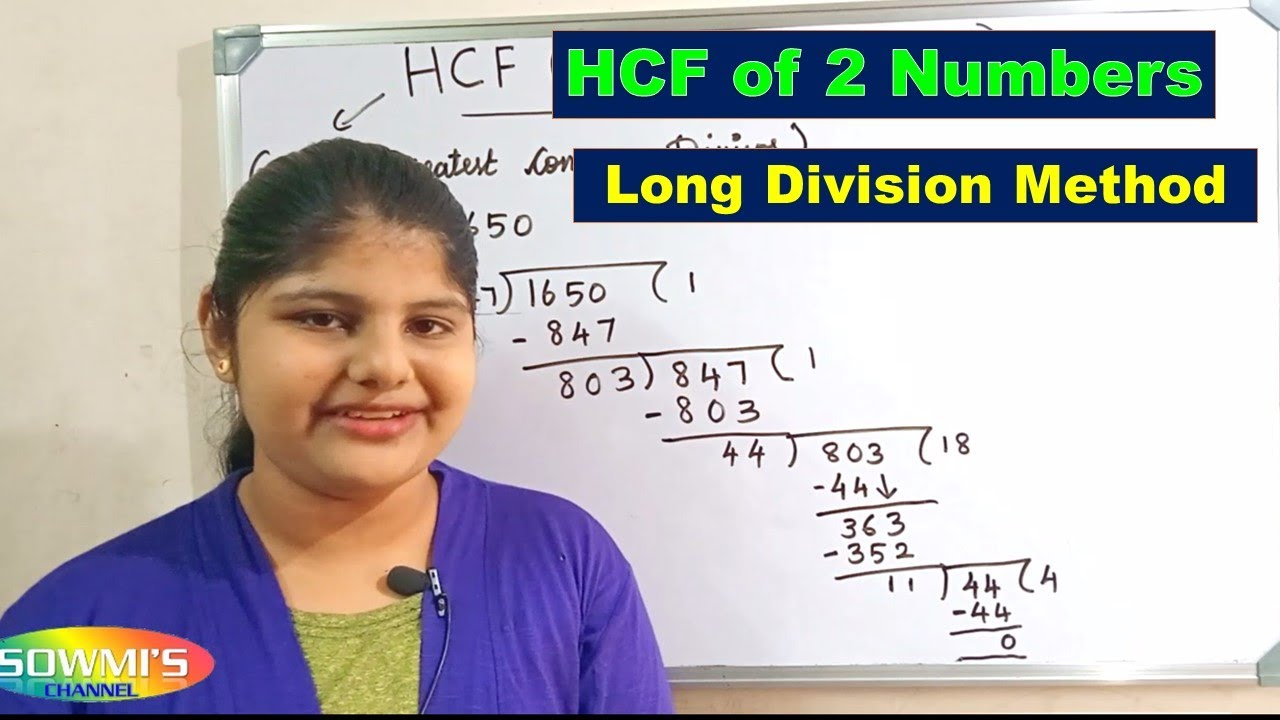# Hcf By Long Division Method## What is HCF by Long Division Method?

The Highest Common Factor (HCF) is the largest number that divides two or more numbers without any remainder. HCF by Long Division Method is a mathematical approach to finding the highest common factor for two or more given numbers. This is a time-consuming method and is mostly used for larger numbers. It is important to understand the concept of multiplication, division, and divisibility of numbers before attempting to solve an HCF by Long Division Method problem.

### Steps to Finding HCF by Long Division Method

The steps to finding HCF by Long Division Method are as follows: 1. Identify the two given numbers and arrange them in descending order. 2. Divide the larger number by the smaller number and find the remainder. 3. Divide the smaller number by the remainder and find the remainder. 4. Repeat the process until the remainder is zero. 5. The last divisor is the highest common factor (HCF).

#### Example of HCF by Long Division Method

Let’s take a look at an example of the HCF by Long Division Method. Suppose we have two numbers, 24 and 36. 1. The larger number is 36 and the smaller number is 24. 2. Divide 36 by 24 and the remainder is 12. 3. Divide 24 by 12 and the remainder is 0. 4. The last divisor is 12 and hence, 12 is the HCF of 24 and 36.

#### Benefits of Using the HCF by Long Division Method

The HCF by Long Division Method is beneficial for a few reasons. First, it is a useful tool for problem-solving and understanding mathematical concepts. This method is also useful for solving problems with larger numbers and can be used for checking the accuracy of other methods used for finding the HCF.

#### Drawbacks of Using the HCF by Long Division Method

The major drawback of using the HCF by Long Division Method is that it can be time-consuming and tedious. This method is better suited for larger numbers and is not the most efficient way to find the HCF when dealing with smaller numbers.

#### Conclusion

The HCF by Long Division Method is a mathematical approach used to find the highest common factor of two or more numbers. Although this method can be time-consuming, it can be beneficial for understanding mathematical concepts and solving problems with larger numbers. It is important to understand the concept of multiplication, division, and divisibility of numbers before attempting to solve an HCF by Long Division Method problem.